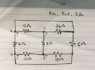# Resistor Network analysis

Eucliwood
Homework Statement:
Finding the resistance when probed at point bc, cd and da
Relevant Equations:
Series and Parallel resistance equation derived from kirchhoff's law with application of ohm's law
Problem Statement: Finding the resistance when probed at point bc, cd and da
Relevant Equations: Series and Parallel resistance equation derived from kirchhoff's law with application of ohm's law

I genuinely don't know what to do on this one. The example our professor made isn't exactly clear and I haven't find any online solution. I just wanted to know how would one calculate the value from those points.

#### Attachments

•59498504_592447804600815_4296939557867225088_n.jpg
13.6 KB · Views: 139

Gold Member
2022 Award
First of all, it's rude to post your figures sideways.

This is mainly a problem in learning to redraw circuits so as to recognize where you can combine elements. If you haven't yet learned how to do that, this problem is going to be tough.

What do you notice about the 10 ohm and 15 ohm resistors?

Eucliwood
A node is a point wherein 3 or more wires constitutes it and the connection is in parallel. I don't know what point to look. But, if i were look at point d the 10 and 15 ohm resistors are connected in parallel. Sorry for the image.

Eucliwood
I'd be glad if you were to include an example in resistors configuration with nodes on it. If it weren't much of a trouble. I cannot find a website that teaches network analysis with nodes embedded.

Gold Member
2022 Award
First of all, it's rude to post your figures sideways.

This is mainly a problem in learning to redraw circuits so as to recognize where you can combine elements. If you haven't yet learned how to do that, this problem is going to be tough.
A node is a point wherein 3 or more wires constitutes it and the connection is in parallel. I don't know what point to look. But, if i were look at point d the 10 and 15 ohm resistors are connected in parallel. Sorry for the image.
Since the 10 and 15 ohm resistors are in parallel, don't you think that would be a good first step in simplifying the circuit?

Eucliwood
First of all, it's rude to post your figures sideways.

This is mainly a problem in learning to redraw circuits so as to recognize where you can combine elements. If you haven't yet learned how to do that, this problem is going to be tough.

Since the 10 and 15 ohm resistors are in parallel, don't you think that would be a good first step in simplifying the circuit?

#### Attachments

willem2
So is this right for Rab?
No. There's a direct path from a to b and and a path that goes through c and d, that's parallel to the direct path.

I would redraw the circuit first with only the points a,b,c,d in it. and replacing all parallel resistances between those points with the equivalent resistance. You can than use that to compute the 3 resistances needed.